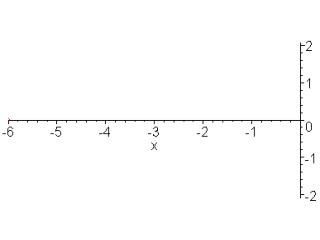Download PresentationStringed instruments are stimulated near one end to enhance the production of harmonics.

# Stringed instruments are stimulated near one end to enhance the production of harmonics. - PowerPoint PPT PresentationDownload Presentation## Stringed instruments are stimulated near one end to enhance the production of harmonics.

- - - - - - - - - - - - - - - - - - - - - - - - - - - E N D - - - - - - - - - - - - - - - - - - - - - - - - - - -
##### Presentation Transcript

1. A taut wire or string that vibrates as a single unit produces its lowest frequency, called its fundamental.

2. A louder sound is produced if the vibrations are transferred to a larger surface (an impedance transformer).

3. Sonometer - a device for studying the properties of vibrating strings and the sounds they produce.

4. The frequency of the vibrating air molecules is the same as the frequency of the vibrating string.

5. The fundamental and the vibrational modes having frequencies that are whole number multiples of the fundamental are called harmonics.

6. The fundamental is the first harmonic. A vibration having a frequency twice that is the second harmonic. Three times that is the third harmonic.

7. Quality, or timbre makes sounds produced by different instruments sound different even when they are producing the same tone with equal intensity.

8. The quality of a sound depends on the number of harmonics produced and their relative intensities.

9. Stringed instruments are stimulated near one end to enhance the production of harmonics.

10. The frequency of a vibrating string is determined by its length, diameter, tension, and density.

11. 1. Law of lengths - the frequency of a string is inversely proportional to its length if all other factors are constant. f / f’ = l’ / l

12. 2. Law of diameters the frequency of a string is inversely proportional to its diameter if all other factors are constant. f / f’ = d’/ d

13. 3. Law of tensions -the frequency of a string is directly proportional to the square root of the tension on the string if all other factors are constant. f / f’ = √ F / √ F’

14. 4. Law of densities - the frequency of a string is inversely proportional to the square root of its density if all other factors are constant. f / f’ = √ D’ / √ D

15. A violin string is 0.035 m long and is stretched with a tension of 27 N, so that it vibrates with a frequency of 256 Hz. What is the frequency when the length is 0.030 m and the tension is 32 N?

16. The length of a closed tube is approximately 1/4 the wavelength of its fundamental resonant frequency. λ ~ 4lλ = 4(l + 0.4d)λ is the wavelength of the fundamental resonant frequency,l is the length of the closed tube, and d is its diameter

17. A closed tube is resonant at odd quarter-wavelength intervals. The resonant frequencies of a closed tube are harmonics, but only odd harmonics of the fundamental.

18. Normal oscillations of air columns are characterized by:1. a displacement node at a closed end, and2. a displacement loop at an open end.

19. The length of an open tube is approximately 1/2 the wavelength of its fundamental frequency.λ ~ 2l λ = 2(l + 0.8d)λ is the wavelength of the fundamental resonant frequency,l is the length of the closed tube, and d is its diameter

20. The resonant frequencies of an open tube are harmonics, and all harmonics of the fundamental mode are present.

21. Two wave trains traveling in the same direction will cause a superposition at a given point whose amplitude varies with time.

22. These amplitude pulsations are called beats.

23. The number of beats per second equals the difference between the frequencies of the component waves.

24. The average frequency is 1/2 the sum of the two frequencies.

25. http://www.ipodphysics.com/wave-harmonic.php